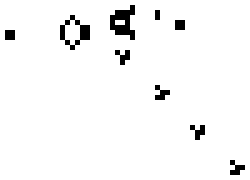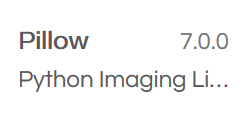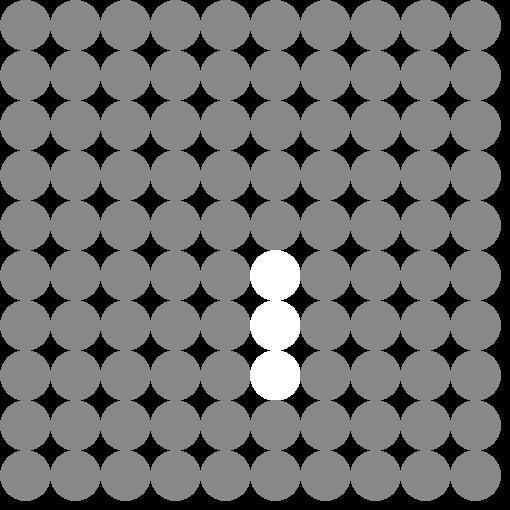# whydoitweet

## Small Sims (3): Simulating living populationsPreviously in Small Sims we looked at simulating people walking in random directions, and boring topics such as simulating crypto prices. Today we are going to do something quite simple and fundamental: simulating living populations

Like last time, we'll use repl.it to make this simulation, because it works just about anywhere.

So our mission is:

• to make a simple simulation that mimics the growth, migration and decay of populations.

As before you can choose to either:

1. Follow it as an actual tutorial, then create a new repl, and add code to your main.py file whenever you see blocks like:

`#This is an example comment.`

2. or simply read/skim/browse the post, and download a working repl.it example at the end, which you can test and modify as you like.

## What libraries do we need today?

We are going to need a few more libraries than usual today. These include the Python Image Library (or its derivative pillow), as well as some routines from the common numpy and scipy libraries. As a whole, our imports look like this:

`from PIL import Image, ImageDraw`

`import numpy as np`

`from scipy.signal import convolve2d`

(update Jan 2020:) To actually use PIL, you also need to set up Pillow, which you can do as follows:

1. Click on the Package Icon on the left side of your screen when you have you repl.it open:2. And then select the Pillow 7.0.0 package, and press + (once you've pressed it, it will change to a -You may have to wait a little while before the package is set up, but you only have to do this once :).

## The Game of Life algorithm

This particular topic happens to map very nicely to a classic cellular automaton model called “the Game of Life”. The Game of Life was devised by John Horton Conway (1937-2020, may he rest in piece), and is arguably more of a simulation than a game, since there are no actual players in it. It consists of a grid of “living” and “dead” cells (which we name life_grid). Here a cell is set to 0 if it is dead, or 1 if it is alive. Whether a cell changes from living to dead (or vice versa), depends on how many living neighbors it has:

• A living cell with two or three neighbors stays alive; it dies due to starvation if it has <2 neighbors, or due to overpopulation if it has >3 neighbors.
• A dead cell with exactly three neighbors becomes alive due to reproduction, and stays dead in all other cases.

When we put these rules in code, it looks roughly like this:

`def step(life_grid):`

`# Count the number of neighbours for each cell.`

`kernel = np.array([[1,1,1],[1,0,1],[1,1,1]])`

`neigh_counts = convolve2d(life_grid,kernel,'same')`

`for x in range(0, life_grid.shape):`

`for y in range(0, life_grid.shape):`

`# Cell is alive`

`if life_grid[x][y] == 1:`

`if neigh_counts[x][y] == 2:`

`pass`

`elif neigh_counts[x][y] == 3:`

`pass`

`else:`

`life_grid[x][y] = 0`

`# Cell is dead`

`else:`

`if neigh_counts[x][y] == 3:`

`life_grid[x][y] = 1`

By the way, the Game of Life is well-known, and you can find a pretty good overview of it on Wikipedia, among many other places.

## Drawing the life grid

Now this life_grid may be interesting, but it'd be cool if we can draw it. To do so, we define the following function to draw a grid on an image d:

`def draw_grid(d, life_grid):`

`""" Draws a game of life grid. """`

`for x in range(0, life_grid.shape):`

`for y in range(0, life_grid.shape):`

`if life_grid[x][y] == 1:`

`d.ellipse([x*50,y*50,(x+1)*50,(y+1)*50],fill="#fff")`

`else:`

`d.ellipse([x*50,y*50,(x+1)*50,(y+1)*50],fill="#888")`

Here, we depict every live cell with a white circle, and a dead one with a gray circle.

## Building your simulation

Now that we have two big building blocks in place (our algorithm for advancing time in the simulation, and our algorithm for drawing the grid), we want to bring in the rest.

First, we want to define the main function in our script, and introduce parameters that we can use to adjust the size of the life grid (in x and y dimensions), as well as the number of time steps we want to simulate:

`if __name__ == "__main__":`

`xsize = 10`

`ysize = 10`

`steps = 5`

Since we have many cells that change state at different times, the best way to visualize our output is by making an animation. To do this, we need to make an list containing multiple images, each of which is large enough to fit all the cells. We do this as follows:

`# make a blank image for the text, initialized to transparent text color`

`base = Image.new('RGBA', (xsize*50+10,ysize*50+10), (0,0,0,0))`

`b = [base]`

`d = [ImageDraw.Draw(base)]`

Not unimportant, we also need to construct the life_grid, and ensure a few cells are alive (no interesting behavior occurs if all the cells are dead...).

`life_grid = np.zeros((xsize, ysize), dtype=int)`

`#put an example block`

`life_grid = 1`

`life_grid = 1`

`life_grid = 1`

With all that in place, we can finally set up the main loop in our simulation. In this loop, we do the following at every time step:

• Draw the grid with cells.
• Add a new empty base image to our animation.
• Advance our life_grid system by one time step, using the game of life algorithm.

Now arguably, you can play around with the order of things in this loop, but this setup is very simple, and gives an okayish result. In code it looks like this:

`for i in range(0, steps):`

`draw_grid(d[-1], life_grid)`

`b.append(Image.new('RGBA', (1024,1024), (0,0,0,0)))`

`d.append(ImageDraw.Draw(b[-1]))`

`step(life_grid)`

Now at this point the simulation has run completely, and all the frames of the animation have been stored. The last thing we then have to do, is save the whole thing to an animated gif:

`b.save("test.gif", save_all=True, append_images=b[1:], duration=1000, loop=0)`

Please note that repl.it still has this image writing bug. So: please delete test.gif in the main directory before you (re-)run your script.

Once you have that in place, you get something like this:## Closing thoughts

In this bread-and-butter blog post I showed how you can program your own Game of Life. If you set the right squares in your life grid to alive (1), you can make all sorts of patterns, including:

• Populations that oscillate between different states (like the small example I made).
• Populations that remain stables (e.g., a 2x2 block).
• Populations that disappear (e.g., a 1x1 block).
• Populations that multiply endlessly (e.g., Gosper's Glider Gun).

I appreciate that these simulations are very common, and you can find them easily online. However, I think it is particularly nice for all of you to be able to actually code such things yourself. In addition, it's also great to see just how simple this stuff is: the code, including the animation-building, is only 68 lines (!) in total.

Also, I managed to introduce you to Pillow and simple animation-building through this tutorial. This is handy, because I'm pretty sure I'll be re-using some of that in my future tutorials :).

I won't leave expert questions this time (there's little I can ask to which Google doesn't know the answer), but I will give you an overview of the full code (and a link to the repl I made). For the Coil subscribers, I will give an update on that other track I have been planning (on the step-by-step building of a computer game).

(Credit: header image courtesy of Wikipedia user Kieff)

Previous: Crypto Price Chart.

## Appendix 1: Code summary

The full code will look like this:

`from PIL import Image, ImageDraw, ImageFont`

`import numpy as np`

`from scipy.signal import convolve2d`

`def step(life_grid):`

`# Count the number of neighbours for each cell.`

`kernel = np.array([[1,1,1],[1,0,1],[1,1,1]])`

`neigh_counts = convolve2d(life_grid,kernel,'same')`

`for x in range(0, life_grid.shape):`

`for y in range(0, life_grid.shape):`

`# Cell is alive`

`if life_grid[x][y] == 1:`

`if neigh_counts[x][y] == 2:`

`pass`

`elif neigh_counts[x][y] == 3:`

`pass`

`else:`

`life_grid[x][y] = 0`

`# Cell is dead`

`else:`

`if neigh_counts[x][y] == 3:`

`life_grid[x][y] = 1`

`def draw_grid(d, life_grid):`

`"""`

`Draws a game of life grid.`

`"""`

`for x in range(0, life_grid.shape):`

`for y in range(0, life_grid.shape):`

`if life_grid[x][y] == 1:`

`d.ellipse([x*50,y*50,(x+1)*50,(y+1)*50],fill="#fff")`

`else:`

`d.ellipse([x*50,y*50,(x+1)*50,(y+1)*50],fill="#888")`

`if __name__ == "__main__":`

`xsize = 10`

`ysize = 10`

`steps = 5`

`# make a blank image for the text, initialized to transparent text color`

`base = Image.new('RGBA', (xsize*50+10,ysize*50+10), (0,0,0,0))`

`b = [base]`

`d = [ImageDraw.Draw(base)]`

`life_grid = np.zeros((xsize, ysize), dtype=int)`

`#put an example block`

`life_grid = 1`

`life_grid = 1`

`life_grid = 1`

`for i in range(0, steps):`

`draw_grid(d[-1], life_grid)`

`b.append(Image.new('RGBA', (1024,1024), (0,0,0,0)))`

`d.append(ImageDraw.Draw(b[-1]))`

`step(life_grid)`

`b.save("test.gif", save_all=True, append_images=b[1:], duration=100, loop=0)`

And a working repl.it of it can be found here (please do make sure you delete test.gif before re-running the script).

Continue reading with a Coil membership.